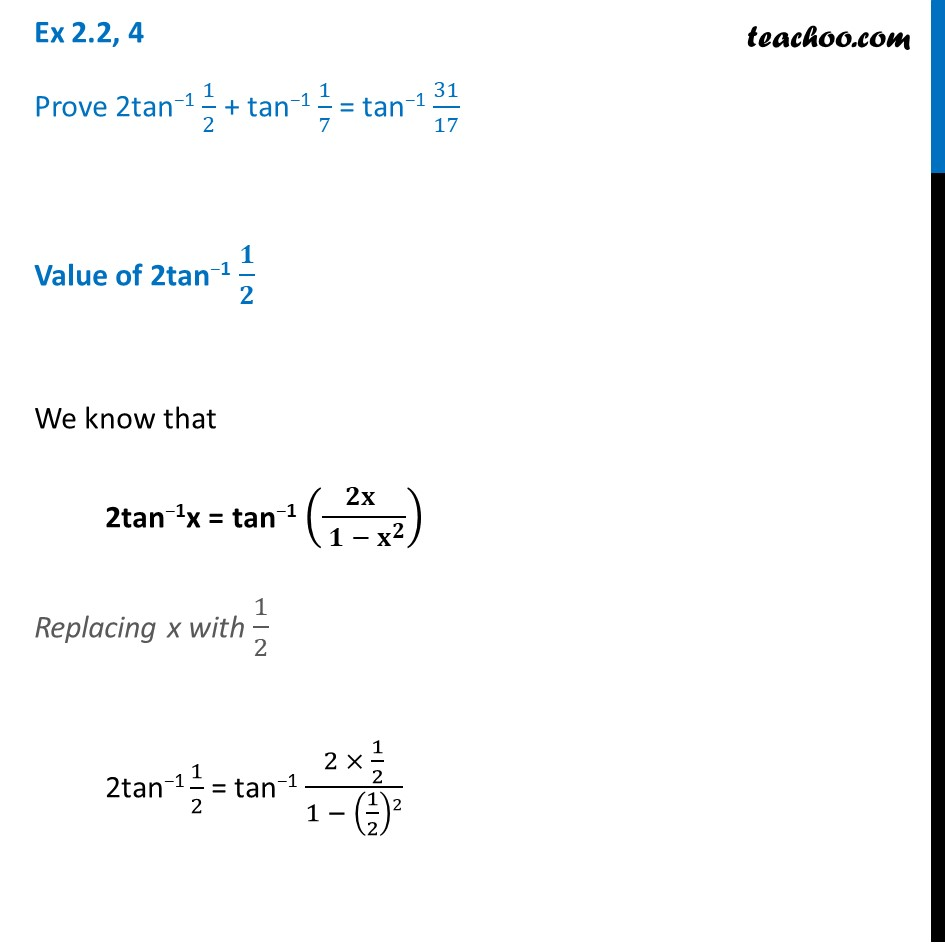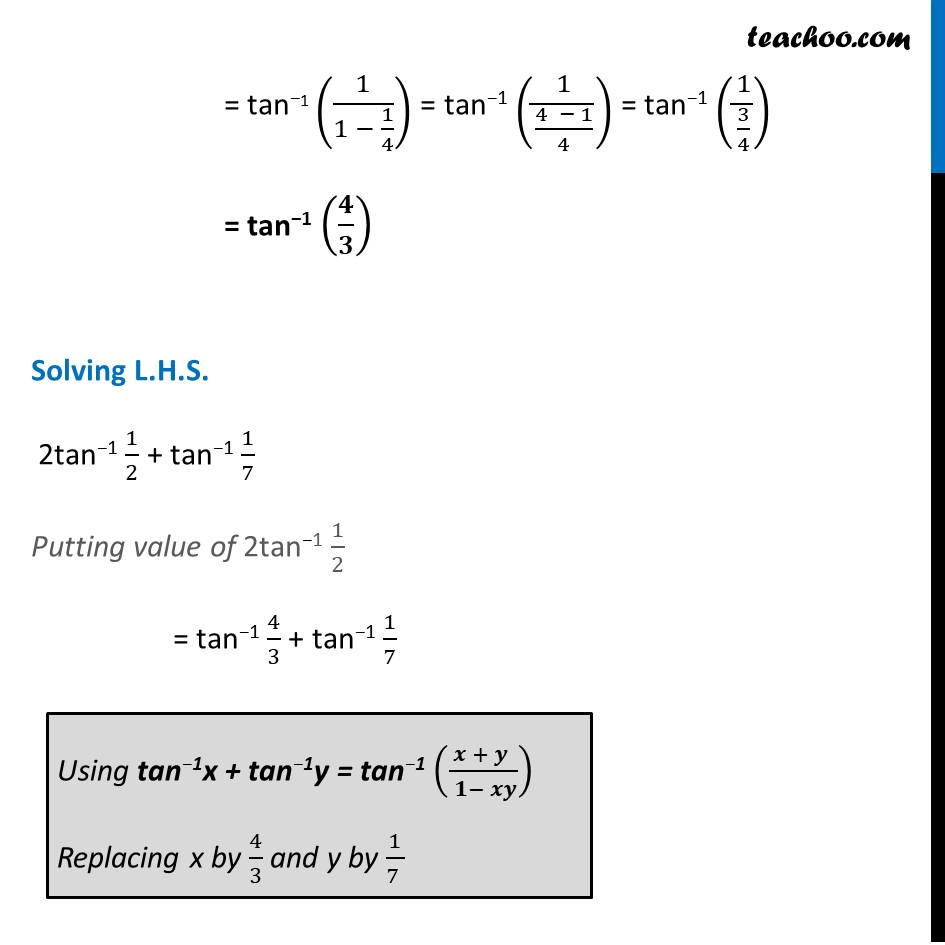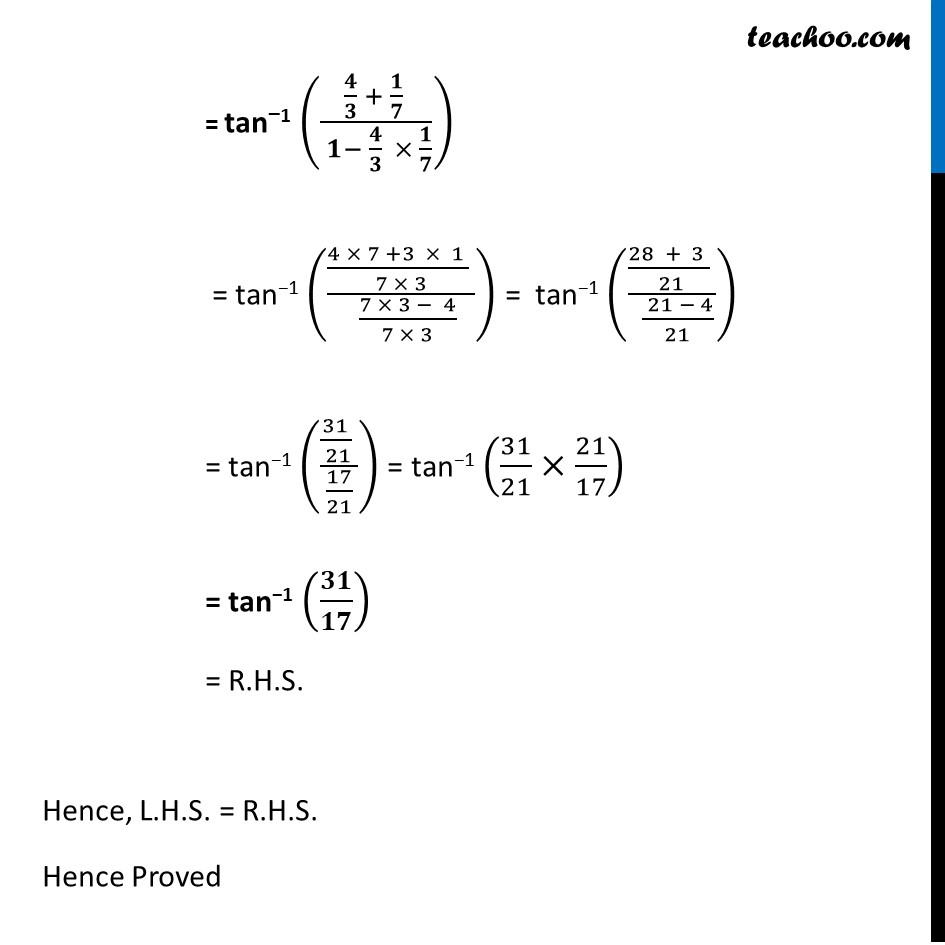Ex 2.2

Chapter 2 Class 12 Inverse Trigonometric Functions
Serial order wiseLearn in your speed, with individual attention - Teachoo Maths 1-on-1 Class

### Transcript

Question 2 Prove 2tan−1 1/2 + tan−1 1/7 = tan−1 31/17 Value of 2tan−1 𝟏/𝟐 We know that 2tan−1x = tan−1 ((𝟐𝐱 )/( 𝟏 − 𝐱^𝟐 )) Replacing x with 1/2 2tan−1 1/2 = tan−1 (2 × 1/2)/(1 − (1/2)2) = tan−1 (1/(1 − 1/4)) = tan−1 (1/((4 − 1)/4)) = tan−1 (1/(3/4)) = tan−1 (𝟒/𝟑) Solving L.H.S. 2tan−1 1/2 + tan−1 1/7 Putting value of 2tan−1 1/2 = tan−1 4/3 + tan−1 1/7 = tan−1 (1/(1 − 1/4)) = tan−1 (1/((4 − 1)/4)) = tan−1 (1/(3/4)) = tan−1 (𝟒/𝟑) Solving L.H.S. 2tan−1 1/2 + tan−1 1/7 Putting value of 2tan−1 1/2 = tan−1 4/3 + tan−1 1/7 Using tan−1x + tan−1y = tan−1 ((𝒙 + 𝒚 )/( 𝟏− 𝒙𝒚)) Replacing x by 4/3 and y by 1/(7 )= tan−1 ((𝟒/𝟑 + 𝟏/𝟕 )/( 𝟏− 𝟒/𝟑 × 𝟏/𝟕)) = tan−1 (((4 × 7 +3 × 1 )/( 7 × 3) )/( (7 × 3 − 4)/(7 × 3))) = tan−1 (((28 + 3 )/( 21) )/( ( 21 − 4)/21)) = tan−1 ((31/( 21) )/(17/21)) = tan−1 (31/21×21/17) = tan−1 (𝟑𝟏/𝟏𝟕) = R.H.S. Hence, L.H.S. = R.H.S. Hence Proved